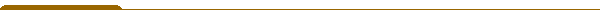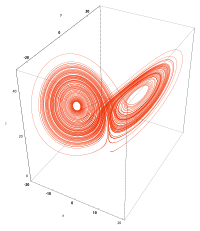Course materials Useful links Jump to: 1MA054   Partiella differentialekvationer, fortsättningskurs (5.0 hp)Lorentz attractor  ©   http://en.wikipedia.org/wiki/Lorenz_attractor Textbooks: [E] Lawrence C. Evans. Partial Differential Equations, AMS, Providence, RI. Series: Graduate Studies in Mathematics, Vol. 19, 1998. [M] Robert C. McOwen, Partial Differential Equations, Methods and Applications, Prentice Hall/Pearson Education, Inc., 2003 (Second Edition) Goals of the course. The course aims at developing the theory for hyperbolic, parabolic, and elliptic partial differential equations in connection with physical problems. Main themes are well-posedness of various initial-value or boundary-value problems, as well as properties of solutions to the wave equation, the heat equation, and the Laplace equation. Contents: Characteristics Classification of second order equations The maximum principle Sobolev spaces Linear elliptic equations Energy methods for Cachy problems for parabolic and hyperbolic equations Systems of conservations laws Instruction: Lectures and problem solving sessions. Examination: Written and, possibly, oral examination at the end of the course. Moreover, compulsory assignments may be given during the course.